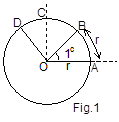×#### Thank you for registering.

One of our academic counsellors will contact you within 1 working day.

Click to Chat

1800-1023-196

+91-120-4616500

CART 0

• 0

MY CART (5)

Use Coupon: CART20 and get 20% off on all online Study Material

ITEM
DETAILS
MRP
DISCOUNT
FINAL PRICE
Total Price: Rs.

There are no items in this cart.
Continue Shopping```Measurment of Angles

Table of Content

Sexagesimal System

Centesimal System

Relationship between Sexagesimal system and Centesimal System

Circular System

Some conversions that shall prove useful in various formulae

Relation between the various systems of measurement

Basic Formulas used in numerical

Related ResourcesTrigonometry is basically a science of measuring angles. An angle is in fact the pivot around which the whole trigonometry revolves.

In general, there are three systems for measuring angles:

Sexagesimal System

Centesimal System

Circular System

Sexagesimal System

This system is also commonly called as the English system. The principal unit of this system is degree (°). A right angle when divided into 90 equal parts and each of the part is called a degree.

This 1° is further divided into 60 equal parts wherein each such part is called a minute denoted by 1’. One minute is further divided into 60 equal parts and each such part is called a second.

These facts can be summarized as:

60 seconds (or 60”) = 1 minute (or 1’)

60 minutes (or 60’) = 1 degree (or 1°)

90 degrees (or 90°) = 1 right angle

Centesimal System

Another important system of measurement of angles is the centesimal system. The principal unit of this system is grade denoted by ‘g’. As in the sexagesimal system, one right angle is divided into 100 equal parts called grades and each grade is further subdivided into 100 minutes and each minute into 100 seconds.

In mathematical form, this can be represented as:

One right angle = 100g

1g = 100 minutes

1 minute = 100 seconds

Relationship between Sexagesimal System and Centesimal System

As explained above,

One right angle = 90 degrees (or 90°) and

One right angle = 100g

From these two equations, it follows that 90° = 100g

Hence, we have 1° = (10/9)g or 1g = (9/10) °

Circular System

This is the most commonly used system of measurement. The unit of measurement used in circular system is radian. One radian, written as 1c is the measure of angle subtended at the centre of the circle by an arc of length equal to the radius of the circle.Consider a circle of radius r, with center at O. Let A be a point on the circle. We take an arc AB on the circle in such a way that its length is equal to the radius of the circle. Then angle AOC is one radian.

We can denote the formula of radian as:

One Radian (1c) = arc length of magnitude ‘r’/ Radius of circle ‘r’

By the above formula it is clear that the ratio is independent of the size of the circle it follows that the radian is a constant quantity.

Watch this Video for more reference

Some conversions that shall prove useful in various formulae

Based on this result, listed below are some of the angles which are generally used in questions:

Relation between the various systems of measurement

D/ 90 = G/100 = 2C/π

Students are advised to learn this formula as questions asked in various exams are often based on the use of this formula.  This formula establishes the relationship between the three systems of measurement.

Basic Formulas used in numerical

Degrees = Radians x 180/ π

Illustration:

Solution:

Since 180° = π, then using the unitary method, we have

180° = π

So, 270° = π/180 . 270

= 3π/2

The equivalent angle is 3π/2.

Illustration:

Solution:

As stated above, Degrees = Radians x 180/ π

Hence, Degrees = π/6 x 180/ π

Illustration:

Convert 43.1025° into minutes and seconds.

Solution:

43° is clearly visible, but we need to convert the remaining part i.e. 0.1025°.

We know that 1° = 60 minutes (or 60’)

Now, 0.1025° = 1025 x 60 = 6.15 minutes

Further, each minute has 60 seconds.

So, 0.15 of a minute = (0.15)(60 /1)

= 9 seconds

Hence, 43.1025° = 43° 6' 9".

Related Resources

Look into the Revision Notes on Trigonometry for a quick revision.

Various Recommended Books of Mathematics are just a click away.

To read more, Buy study materials of Trigonometry comprising study notes, revision notes, video lectures, previous year solved questions etc. Also browse for more study materials on Mathematics here.
```### Course Features

• 731 Video Lectures
• Revision Notes
• Previous Year Papers
• Mind Map
• Study Planner
• NCERT Solutions
• Discussion Forum
• Test paper with Video Solution